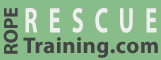# Rope Angles

The angle of ropes that are connected to anchors can have a tremendous affect on forces.

## 0° Angle

This system has a 100-pound load suspended from two anchors. The two ropes are basically parallel which creates an angle of approximately 0°. In this case, each anchor is holding half of the load (i.e., 50 pounds).

(You can mentally replace the word "pounds" with "kilograms" throughout this page.)

## 90° Angle

This system has the same 100-pound load suspended from two anchors, but this time the angle between the two ropes is 90°. In this case, each anchor is holding approximately 70 pounds. Yes, the 100‑pound load is actually generating 140 pounds of force (i.e., 2 x 70 pounds)!

## 150° Angle

As the angle between the two ropes increases, the force on the anchors increases—dramatically. This next system has the same 100-pound load, but this time the angle between the ropes is 150 degrees. The force on each anchor (and on each rope) is now more than 190 pounds—that is almost double the 100-pound load.

## Angles and Forces

Forces by Rope Angle
50 lb.
45° 54 lb.
90° 71 lb.
120° 100 lb.
140° 146 lb.
150° 193 lb.
160° 288 lb.
170° 574 lb.

This table shows the force-multiplying effects of rope angles. The left-hand column is the angle between the ropes and the right hand column is the force that would be applied to each rope and anchor. (Again, you can mentally replace the term "pounds" in this table with "kilograms.")

You can see that when the angle between the ropes is 120 degrees, each rope will receive forces equivalent to the entire load (in this case, 100 pounds). The 120-degree angle is sometimes referred to as the "critical angle" to remind rescuers that exceeding 120 degrees will result in more than 100% of the load being applied to each rope.

Rescuers do not need to memorize this table. Knowing that 50% of the load is on each strand when the ropes are side-by-side (i.e., 0 degrees) and that 100% of the load is on each strand when the ropes are 120 degrees is adequate. As a general rule of thumb, most rescuers ensure that the angle between the ropes never exceeds 90 degrees. The choice of 90 degrees is selected because it is easy for people to visualize and it provides an additional safety margin from the "critical angle" of 120 degrees.

This chart shows how forces increase dramatically as the angles between the ropes increase.

## Handy Protractor

With your thumb spread widely, the angle between your thumb and index finger is approximately 90 degrees (generally considered the maximum angle). When all of your fingers are spread widely, the angle between your thumb and little finger is approximately 120 degrees (the "critical angle").

## Calculator

You can use this calculator to calculate forces that are generated by any load at any angle.

 Load: kilograms pounds Angle between ropes: Exerts xxx xxx force on each rope

## Nerdy Details

So how can the 100-pound load in this 150-degree system generate more than 190 pounds on two anchors? That is a total of ~380 pounds being generated by a single 100-pound load!

In fact, each anchor is still receiving only 50 pounds of vertical force from the 100-pound load. However, the load is also pulling the two anchors horizontally toward each other as shown in this illustration.

The 50 pounds of vertical force, run through a little trigonometry, generates a net diagonal force of 193 pounds.

The following formulas show the math to calculate the net force on each strand of rope. Each strand is holding 50 pounds of vertical force at a 75° angle (one-half of the 150 degrees) which generates ~193 pounds of tension on each strand of rope.

These next formulas show how you can calculate only the horizontal force on each of the anchors if you know the vertical force (50 pounds in our example) and the net force on each strand of rope (193.2 pounds in our example). These formulas (and the above illustration) show that there are 186.6 pounds of horizontal force on each of the anchors. The 186.6 pounds of horizontal force plus the 50 pounds of vertical force results in 193 pounds of total force on each of the anchors.

You do not need to understand the trigonometry that is used to calculate these forces (and vRigger can do this math for you), but every rescuer must know that wide-rope-angles can generate massive forces that can easily exceed the load's weight (e.g., in the above example, the 100-pound load is generating approximately 380 pounds of force).

Try It.
Risk Free.